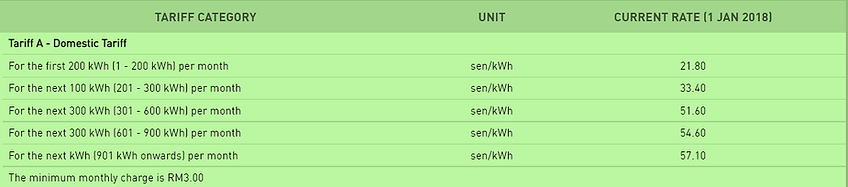top of page

How much Electricity Bill is my Electrical Appliance costing me a month?

This calculator provides an estimation for the electricity cost to operate an electrical appliance based on its average power rating.

The steps to do so are simple and straightforward as follows:Note that depending on Utility Provider, the energy tariff rate may be subject to nature of use and total energy usage.

You can get information on energy tariff rate from your utility provider.

Example below shows the domestic tariff rate for a utility provider in Malaysia. If your total monthly energy consumption exceeds 900 kWh, you should be multiplying your energy consumption with a tariff rate of 57.10 cent/kWhExample:

Given that house A has a total energy consumption >900kWh per month, calculate the estimated electricity cost for the Air Conditioner Unit which averagely operates 8 hours a day, based on the power rating label and the tariff rate below.Solution:bottom of page